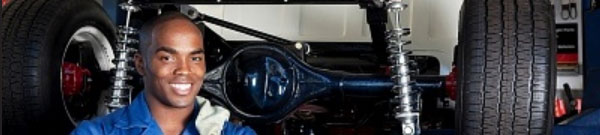# Principle of Torque ConverterTransmission of Torque

When the pump impeller speed increases, centrifugal force causes the fluid to start flowing outward from the center of the pump impeller. As the pump impeller speed rises further, the fluid is forced out away from the pump impeller. The fluid strikes the vanes of the turbine runner, causing the turbine runner to start rotating in the same direction as the pump impeller. Fluid flows inward along the vanes of the turbine runner. When it reaches the interior of the turbine runner, the runner’s curved inner surface redirects the fluid back toward the pump impeller, and the cycle begins again. Transmission of torque is effected by the fluid circulation through the pump impeller and turbine runner.

Torque Multiplication

Toque multiplication by the torque converter is performed by returning the fluid, which still has energy after it has passed through the turbine runner, into the pump impeller by use of the stator vane. In other words, the pump impeller is rotated by the torque from the engine, to which is added the torque of the fluid returning from the turbine runner. That is to say, the pump impeller multiplies the original input torque for transmission to the turbine runner.

Torque Converter Performance

Torque Ratio and Transmission Efficiency

Torque multiplication by the torque converter becomes greater in proportion to the vortex flow. This means that the torque becomes the maximum when the turbine runner is stopped. Torque converter operation is divided into two operating ranges:

The converter range, in which torque multiplication takes place.

The coupling range, in which simple torque transmission but no torque multiplication occurs. The clutch point is the dividing line between these two ranges.

The transmission efficiency of the torque converter indicates how effectively the energy imparted (given) to the pump impeller is transmitted to the turbine runner. The energy here refers to the engine output itself and is proportional to the engine speed (rpm) and torque. Since the torque is transmitted at nearly 1:1 in a fluid coupling, the transmission efficiency in the coupling range increases linearly in proportion to the speed ratio. However, the transmission efficiency of the torque converter does not reach 100%, but is generally approx. 95%. This energy loss is due to the heat generated in the fluid and friction. When the fluid circulates, it is cooled by the oil cooler.